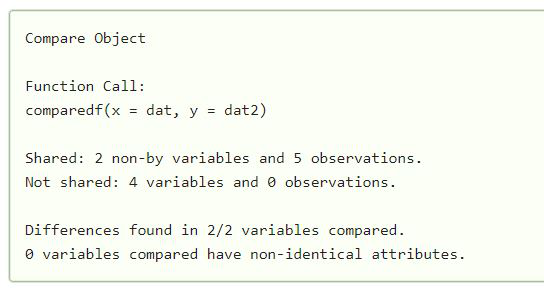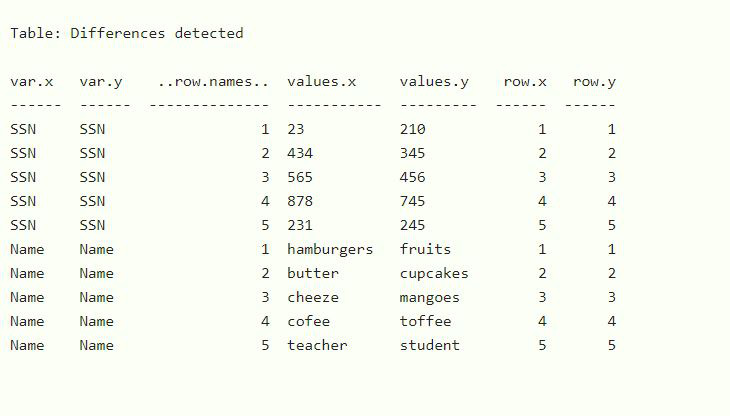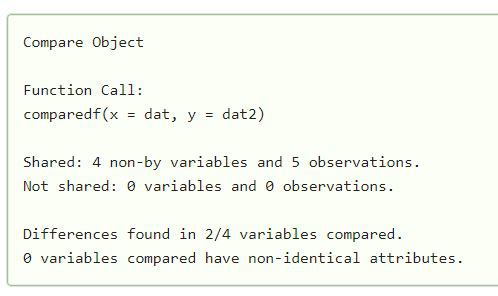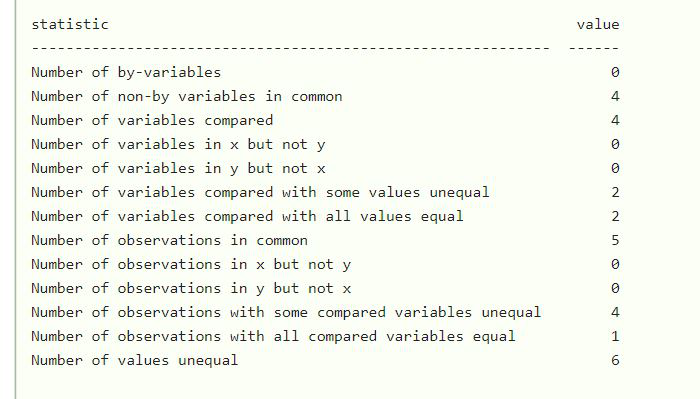Related Articles

# Comparing two data sets in R

• Last Updated : 16 Mar, 2021

There may be a situation where we have to compare datasets to do data analysis, with having the same structure but differences in the data. So to identify what is changed in the dataset and to get a summary to which extent it is changed. We can use the compare package in R. We can easily use this package to compare two data frames and check out the summary of what extent it is changed.

The function comparedf() is used to compare two dataframes in R. The function takes two dataframes and then check them for comparison.

Syntax: comparedf(dataset1,dataset2)

Parameters:

• dataset1, dataset2: These are the two datasets to be compared.

Also, we can see the summary of the difference as:

Syntax:

`summary(comparedf(dat,dat2)`

Approach:

• Import library
• We have to simply create datasets.
• Pass the dataset as a parameter in the comparedf() function.
• It will detect the difference if any.
• Use the summary method to check the complete summary.

Example1 :

## R

 `library``(arsenal)`` ` ` ` `dat <- ``data.frame``(``SSN = ``c``(23,434,565,878,231),  ``Name=``c``(``"hamburgers"``,``"butter"``,``"cheeze"``,``"cofee"``,``"teacher"``),  ``Age = ``c``(7,8,6,43,56),  ``Gender = ``c``(0,1,0,1,0)``)`` ` `dat2 <- ``data.frame``(``SSN = ``c``(210,345,456,745,245),  ``Name=``c``(``"fruits"``,``"cupcakes"``,``"mangoes"``,``"toffee"``,``"student"``),  ``Number= ``c``(3,5,5,6,77),  ``Different = ``c``(0,0,1,1,0)``)`` ` `summary``(``comparedf``(dat,dat2))`

Output:Example 2:

## R

 `library``(arsenal)`` ` ` ` `dat <- ``data.frame``(``Rollnumber = ``c``(1,2,3,4,5),  ``Name=``c``(``"Akash"``,``"John"``,``"Tina"``,``"Kakashi"``,``"Brad"``),  ``Age = ``c``(7,8,6,5,7),  ``Marks = ``c``(78,98,76,67,89)``)`` ` `dat2 <- ``data.frame``(``Rollnumber = ``c``(1,2,3,4,5),  ``Name=``c``(``"Akash"``,``"John"``,``"Tina"``,``"Kakashi"``,``"Brad"``),  ``Age = ``c``(7,9,6,7,8),  ``Marks= ``c``(78,98,56,87,67)``)`` ` `comparedf``(dat,dat2)`

Output:My Personal Notes arrow_drop_up